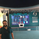# Min/Max Value Multiple Series Function

3287 vues
3287
Trying different solutions to find the minimum/maximum value in a set of predefined seriescoward_
@coward_,

Use this to get exactly value. Since TV is equaling last index value.. So you have to start with "0"

array(index) =>
index==0 ? a : index == 1 ? b :
index == 2 ? c : index == 3 ? d : na

maxf(arrayLen) =>
max = array(0)
for i = 0 to arrayLen-1
max := max(max,array(i))
max
Répondre